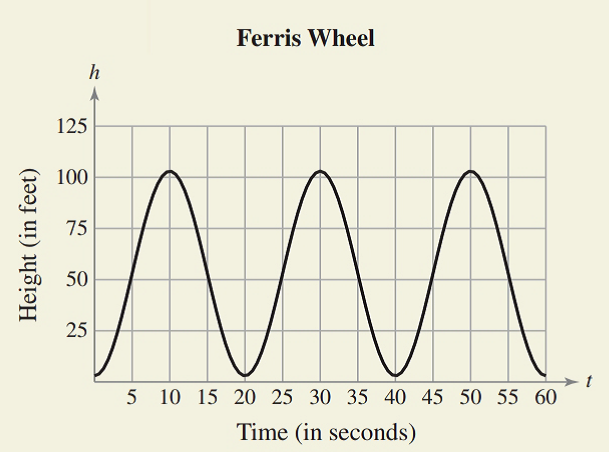Chapter 8.4, Problem 74E### Calculus: An Applied Approach (Min...

10th Edition
Ron Larson
ISBN: 9781305860919

#### Solutions

Chapter
Section### Calculus: An Applied Approach (Min...

10th Edition
Ron Larson
ISBN: 9781305860919
Textbook Problem
1 views

# HOW DO YOU SEE IT? The graph shows the height h (in feet) above ground of a seat on a Ferris wheel at time t (in seconds).(a) What is the period of the model? What does the period tell you about the ride?(b) Find the open intervals on which the height is increasing and decreasing.(c) Estimate the relative extrema in the interval [0. 60].(a)

To determine

The period of the provided model and interpret its meaning. The provided model can be shown as:Explanation

Given Information:

The provided model can be shown as:

Draw the provided model as:

Period is the distance between two consecutive crests. Thus, from above model, period of the model can be calculated as:

T=30 s10

(b)

To determine

The open interval for height is increasing and decreasing with the help of the provided model, which can be shown as:(c)

To determine

The relative extrema in the intervals [0,60] with the help of the provided model, which can be shown as:### Still sussing out bartleby?

Check out a sample textbook solution.

See a sample solution

#### The Solution to Your Study Problems

Bartleby provides explanations to thousands of textbook problems written by our experts, many with advanced degrees!

Get Started

#### Solve the equations in Exercises 126. 2(x21)x2+1x4x2+1x2+1=0

Finite Mathematics and Applied Calculus (MindTap Course List)

#### Graph each equation: y=4x

Elementary Technical Mathematics

#### True or False: converges.

Study Guide for Stewart's Single Variable Calculus: Early Transcendentals, 8th

#### Identify and describe the steps in the research process.

Research Methods for the Behavioral Sciences (MindTap Course List)